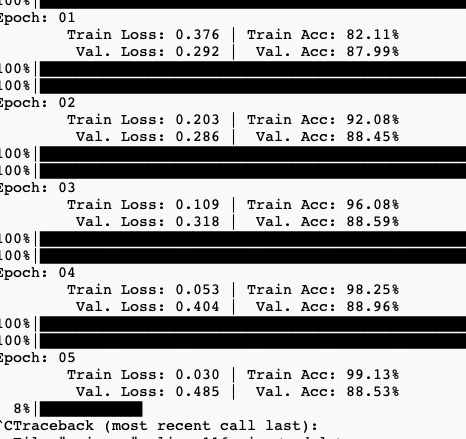# Is that overfitting? IMDB dataset 50K

Hi everyone, when I train my model, the result looks like in this picture.I think this is not right, since the best epoch is 2 and but in epoch 3 valid loss is increase. I think it is overfitting.
here is my code
‘’’
class BERTGRUSentiment(nn.Module):
def init(self,bert,hidden_dim,output_dim,n_layers,bidirectional,dropout):

``````    super().__init__()

self.bert = bert
self.bert_drop = nn.Dropout(0.3)
self.out = nn.Linear(768, 1)

def forward(self, ids, mask, token_type_ids):
o2 = self.bert(ids, attention_mask=mask, token_type_ids=token_type_ids)
bo = self.bert_drop(o2)
output = self.out(bo)
return output
``````

model.to(device)
criterion = nn.BCEWithLogitsLoss()
criterion = criterion.to(device)
# optimizer = optim.Adam(model.parameters())
param_optimizer = list(model.named_parameters())
no_decay = [“bias”, “LayerNorm.bias”, “LayerNorm.weight”]
optimizer_parameters = [
{
“params”: [
p for n, p in param_optimizer if not any(nd in n for nd in no_decay)
],
“weight_decay”: 0.001,
},
{
“params”: [
p for n, p in param_optimizer if any(nd in n for nd in no_decay)
],
“weight_decay”: 0.0,
},
]

``````num_train_steps = int(len(train_dataset) / batch_size * config.EPOCHS)
optimizer = AdamW(optimizer_parameters, lr=3e-5)
scheduler = get_linear_schedule_with_warmup(
optimizer, num_warmup_steps=0, num_training_steps=num_train_steps
)
``````

‘’’’
can you give me some ideas about it?

Yes, it seems your model is overfitting and the general recommendation would be to add more regularization to the model e.g. via dropout etc.

PS: you might want to change the title to get better support, as it’s a bit ambiguous at the momenthaha, thanks very much,